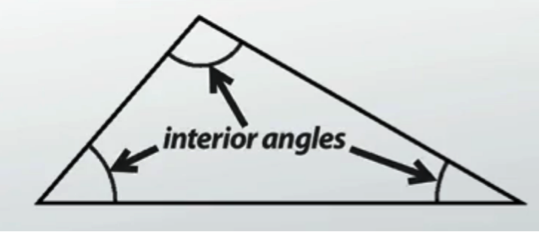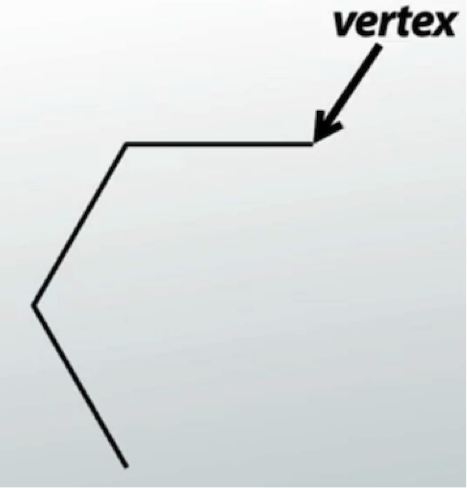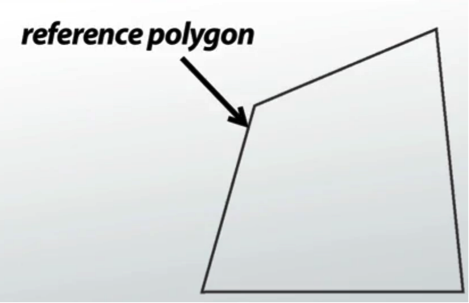# Constructing Similar Polygons

An error occurred trying to load this video.

Try refreshing the page, or contact customer support.

Coming up next: Properties of Right Triangles: Theorems & Proofs

### You're on a roll. Keep up the good work!

Replay
Your next lesson will play in 10 seconds
• 0:01 Defining & Identifying…
• 1:19 Procedure for…
• 3:40 Variations of Similar Polygons
• 4:09 Lesson Summary

Want to watch this again later?

Timeline
Autoplay
Autoplay
Speed

#### Recommended Lessons and Courses for You

Lesson Transcript
Instructor: Artem Cheprasov
This lesson will teach you how to construct similar polygons, which are very useful in many quantitative fields. You'll learn that all of this has something to do with, of all things, snowflakes!

## Defining and Identifying Similar Polygons

Imagine you're outside on a cold winter day with snow falling from the sky and you catch two identically shaped snowflakes, with one of them being twice as large as the other. Observe that while each edge of the larger snowflake is proportionally longer than its corresponding edge in the smaller snowflake. All of the corresponding angles are the same. This is just one real-life example of similar polygons. In case you're already lost with all the technical mumbo jumbo, let's define two important terms:

1. Congruent means equal, as in congruent angles, meaning angles that are equal to each other.
2. A polygon is a closed, flat figure with three or more straight sides..

Here are some examples of polygons that should look familiar:What makes one polygon similar to another? Two polygons are similar if and only if their vertices could be paired up, such that:

1. The ratio of the lengths of corresponding sides is the same
2. All corresponding interior angles are congruent

By interior angles, this means angles that are inside the polygon, as shown on the screen.So how do we construct similar polygons?

## Procedure for Constructing Similar Polygons

You can construct similar polygons by following these steps:

1. You need to have a reference polygon. Any polygon can serve as a reference as long as it is a 2-dimensional geometric shape with three or more straight sides.
2. The to-be-constructed similar polygon has to follow the definition of what it means to be similar (namely, the ratio of all lengths of corresponding sides is the same, all corresponding interior angles are congruent). Decide what you want this ratio of corresponding sites to be. You're now ready to draw the new polygon.
3. Using a ruler and a protractor, draw a point and two emanating line segments that have a corresponding vertex in the reference figure. The angle in the new figure should be the same as it corresponding angle in the reference polygon and the two line segments should be sized based on the ratio from step two.
4. Each of your new line segments should lead to another vertex, as in the figure on the screen.Repeat step three until the new similar polygon is complete.

As an example, consider the quadrilateral shown on the screen as our reference.If we decide that the ratio of corresponding sides is equal to 2, the resulting similar polygon can be constructed as follows:

To unlock this lesson you must be a Study.com Member.

### Register to view this lesson

Are you a student or a teacher?

### Unlock Your Education

#### See for yourself why 30 million people use Study.com

##### Become a Study.com member and start learning now.
Back
What teachers are saying about Study.com

### Earning College Credit

Did you know… We have over 160 college courses that prepare you to earn credit by exam that is accepted by over 1,500 colleges and universities. You can test out of the first two years of college and save thousands off your degree. Anyone can earn credit-by-exam regardless of age or education level.